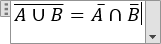# How to insert a Boolean algebra equation

Word
This tip shows how to insert a Boolean algebra equation, for example, one of De Morgan's laws.

How to add an equation in your document, see Working with Microsoft Equation.

To add operators of the Boolean algebra, do the following:

## In the Professional format:

2.   On the Equation tab, in the Structures group, click the Accent button: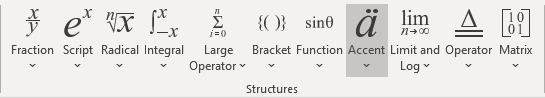In the Accent list, choose Bar: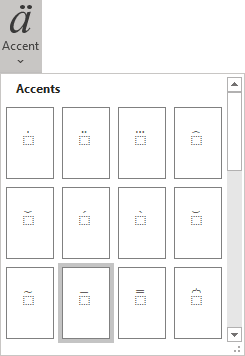3.   In the box:

3.1.   Enter A.

3.2.   On the Equation tab, in the Symbols group, in the list of symbols, choose Union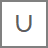.

3.3.   Enter B.

4.   Then enter =.

5.   On the Equation tab, in the Structures group, click the Accent button and then choose Bar. In the box, enter A.

6.   On the Equation tab, in the Symbols group, in the list of symbols, choose Intersection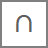.

7.   On the Equation tab, in the Structures group, click the Accent button and then choose Bar. In the box, enter B :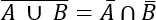## In the Linear format:

2.   Enter (A \cup. Then you enter a space key, this linear formula transformed to the professional format: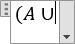3.   Enter B) \bar and then a space bar: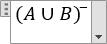4.   Enter =: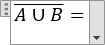5.   Enter A \bar \cap B \bar. Then you enter a space key, your linear formula transformed to the professional format: